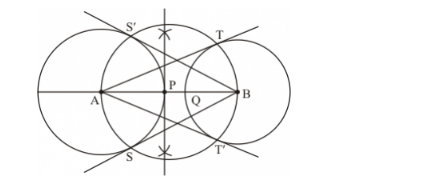# Draw a line segment AB of length 8 cm. Taking A as centre, draw a circle of radius 4 cm

Question:

Draw a line segment AB of length 8 cm. Taking A as centre, draw a circle of radius 4 cm and taking B as centre, draw another circle of radius 3 cm. Construct tangents to each circle from the centre of the other circle.

Solution:

Given that

Construct a circle of radius, andextended diameter each at distance of 7cm from its centre. Construct the pair of tangents to the circle from these two points.

We follow the following steps to construct the givenStep of construction

Step: I First of all we draw a line.

Step: II taking as a centre and draw a circle of radius. Similarly, taking as a centre and draw a circle of radius.

Step: III draw the perpendicular bisector ofStep IV : draw the another circle with taking the bisector point as centre and radius = mid point ofwhich cut the pointStep: V joinsandrespectively.as well asto obtain the require tangents.

Thus,are the required tangents.